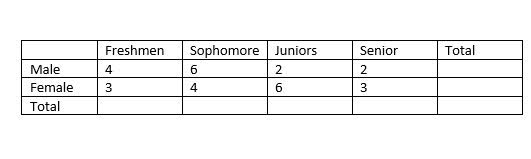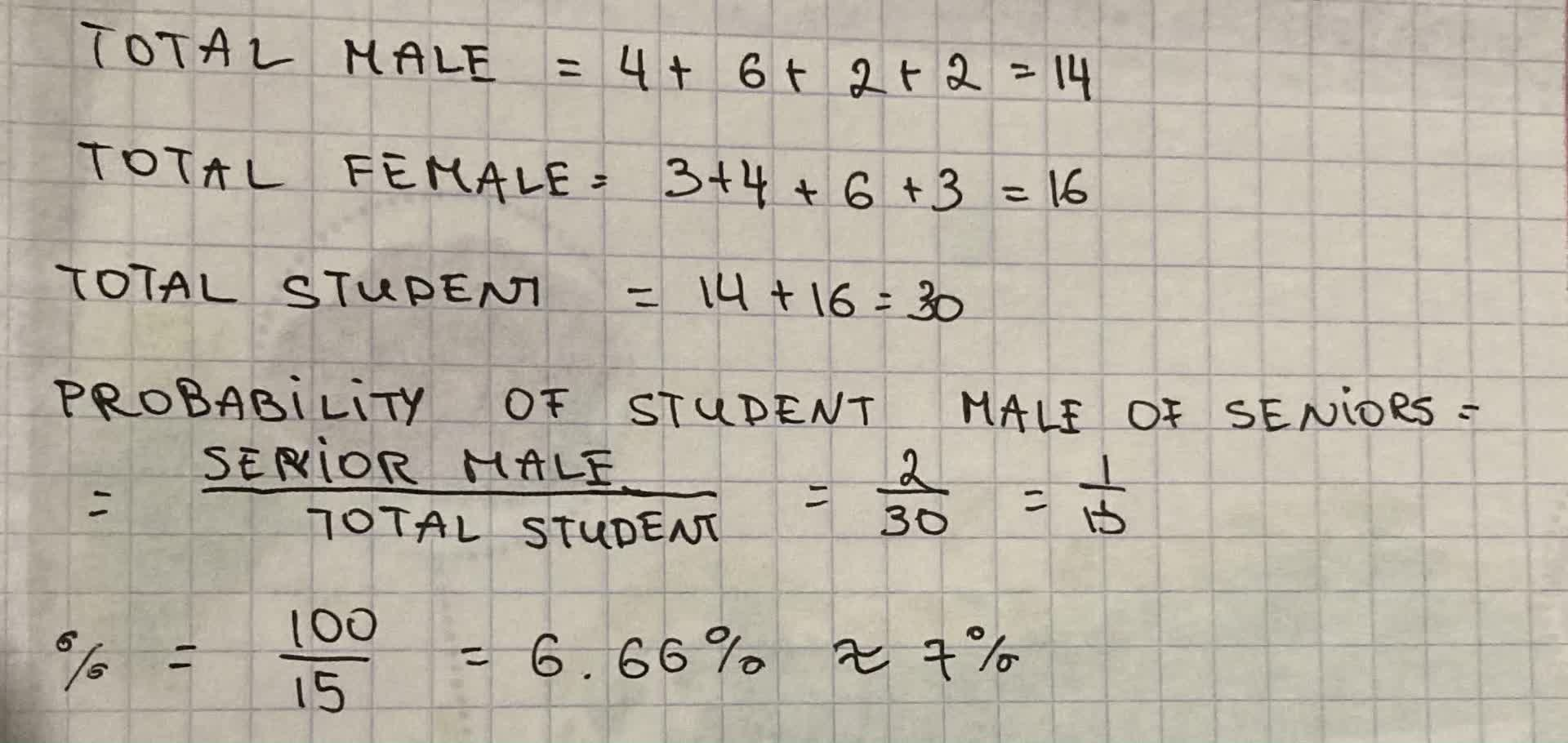If a student is selected at random, find the probability the student is a male given that it’s a senior. Use the two-way table belowRivka Thorpe 2021-09-16 Answered

If a student is selected at random, find the probability the student is a male given that it’s a senior. Use the two-way table below.Want to know more about Two-way tables?

• Questions are typically answered in as fast as 30 minutes

Solve your problem for the price of one coffee

• Math expert for every subject
• Pay only if we can solve itLatisha Oneil

Calculating the probability: